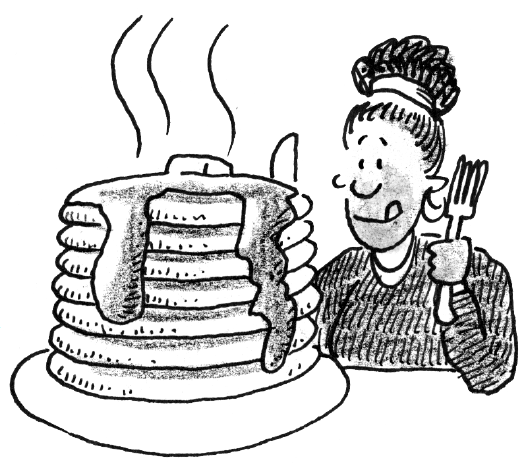### Home > APCALC > Chapter 1 > Lesson 1.4.4 > Problem1-182

1-182.

Marni loves pancakes and likes to eat them in a tall stack. Assume that she always makes $8$ pancakes each with a thickness of $\frac { 1 } { 4 }$ inch.

1. Last Saturday, Marni decided to make square pancakes. If the largest pancake had an edge of $9$ inches and each pancake had an edge $\frac { 1 } { 4 }$ inch shorter than the one below it, calculate the volume of pancakes Marni ate last Saturday.

$\text{Volume} = \left(\text{Area of Base}\right)\left(\text{Thickness}\right)$

The area of the base of each pancake is the edge squared.

$132.6875$ in²

2. Next Sunday, Marni will make circular pancakes. Each pancake will have a diameter $1$ inch smaller than the one below it and the smallest pancake will have a radius of $2$ inches. How much volume is Marni planning to eat?

$\text{In this case, the area of the base of each pancake }\pi r^2.\text{ Thickness remains } \frac{1}{4}.$